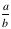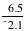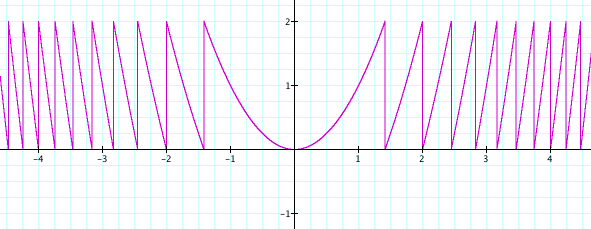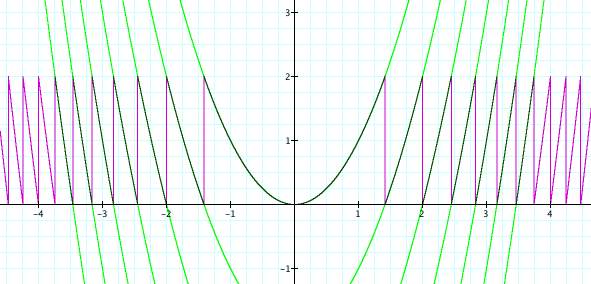# Conceptions of Function Definitions

## Action vs. Process conceptions of formulae

Harel and Dubinsky (1991) pointed out two very different conceptions of function definitions held by people. One, an action conception, is a typified by a person looking at a formula as a prescription for calculating, a "command to calculate", so to speak. Students holding this view imagine function values being calculated, laborously, one at a time. Students holding an action conception of a formula cannot envision a graph as emerging from two quantities covarying simultaneously, nor can they see it as a mapping from one set of values to another set of values.

Another conception of formulae, a process conception, is typified by a person looking at a formula as "self evaluating". It evaluates itself. We give it a number and, bang, we get a number back. This does not mean that the person can, in fact, perform calculations instantaneously. Rather, he or she envisions the formula as giving results instantaneously.

How do students develop process conceptions of formulae? By experiencing supportive instruction that persistently employs the mantra, "variables vary, go slow!".

## Activity

During class we went through several activities, including this one, where you had to first build an action conception of a formula, then we built a process conception of the same formula by employing "variables vary, go slow!" As a reminder, here is the activity we did.

We normally think that b and a in "a mod b" stand for whole numbers. 27 mod 3 is 0, because 27 ÷ 3 has remainder 0. 27 mod 5 is 2, because 27 ÷ 5 has remainder 2. But we can think generalize this idea to fractions and irrational numbers, too. The definition of "b mod a" that does this is:

(a mod b) is the remainder obtained when subtracting mb from a, where m is the largest integer less than or equal to.

By this definition, (6.5 mod 2.1) = 0.2, since 3 is the greatest integer less than or equal to, and 6.5 - (3)(2.1) = 0.2. Similarly, (6.5 mod -2.1) = -1.9 because –4 is the largest integer less than or equal toand 6.5 – (-4)(-2.1) = -1.9 (you should determine this for yourself).

In class, we spent a considerable amount of time wherein you practiced how to calculate (a mod b) for various values of a and b. After calculating many values, you came to the realization that the result would always be positive when the b (the modulus) is positive, and that the result will always be negative when the modulus is negative. We then went through the example of "what will the graph of y = mod(x2,2) look like?" Again, we employed the mantra "variables vary, go slow!" to figure out that the graph will look like this:We also reasoned that the graph's overall behavior is like y=x2 for x being between 0 and square √2, like y=x2-2 for x between √2 and 2, like y=x2-4 for x between 2 and √6, etc. We checked this by graphing each of these functions on top of the graph of y = mod(x2,2), getting this:At each stage, we explained why the graphs behave as they do, and our explanations were rooted in the definition of mod(a,b) in conjunction with the idea that as x varies the value of mod(x2,2) varies.

## Extension

Given that a mod b is defined as above, do this for the first four functions in the following list: (a) predict its behavior, giving your reasoning behind your prediction; (b) explain its actual behavior. (We did f1 in class. You can repeat it for practice if you wish, but it is not mandatory.) Please consider the last one as a challenge question.

f1(x) = mod(x2,2)

f2(x) = x + mod(x2,2)

f3(x) = mod(x3,2)

f4(x) = mod(x2,x)

f5(x) = mod(x2,cos(x))

## Pedagogy

What lessons have you learned from this assignment with regard to how you might help students develop process conceptions of function definitions and covariational understandings of functions?